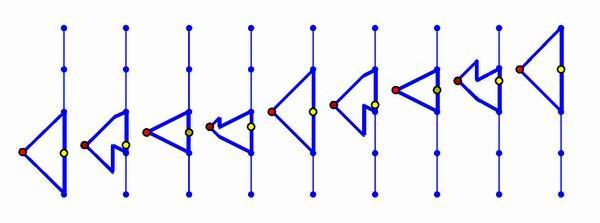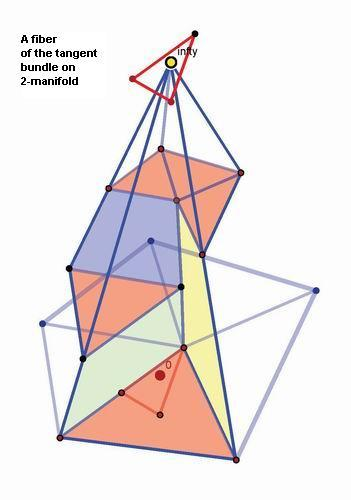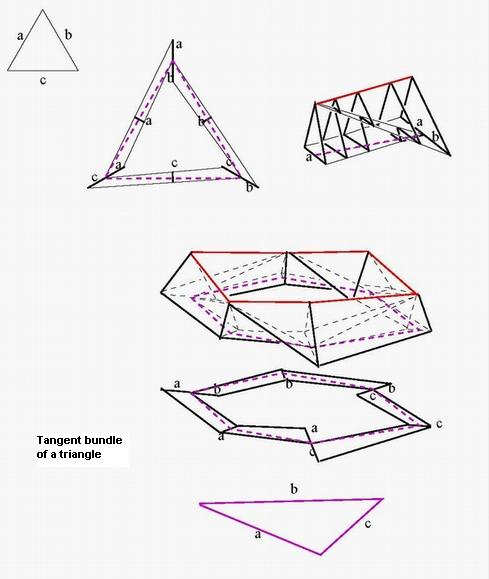fibers of the tangent bundle on 1-manifold

"Combinatorial PL-fiber-bundles and fragmentation of
fiberwise homeomorphism" Preprint PDMI 12-06
Russian. pdf
109 pp.  700 KB version 30.04.07. Published in Zapiski Nauchnyh Seminarov POMI ,v344, p 56-173, 2007. English translation is located in ArXiv arXiv:0708.4039 and published in Journal of Mathematical Sciences Vol 145 No 3, 2007
===========

==============
Abstract
With a compact PL manifold X we associate a category T(X). The objects of T(X) are all combinatorial manifolds of type X, and morphisms are combinatorial assemblies. We prove that the homotopy equivalence BT (X) \approx BPL(X) holds, where PL(X) is the simplicial group of PL-homeomorphisms. Thus the space BT(X) is a canonical countable (as a CW-complex) model of BPL(X). As a result, we obtain functorial pure combinatorial models for PL fiber bundles with fiber X and a PL polyhedron B as the base. Such a model looks like a T(X)-coloring of some triangulation K of B. The vertices of K are colored by objects of T(X) and the arcs are colored by morphisms in such a way that the diagram arising from the 2-skeleton of K is commutative. Comparing with the classical results of geometric topology, we obtain combinatorial models of the real Grassmannian in small dimensions: BT(S^{n-1}) \approx BO(n) for n=1,2,3,4. The result is proved in a sequence of results on similar models of B\PL(X). Special attention is paid to the main noncompact case X=R^n and to the tangent bundle and Gauss functor of a combinatorial manifold. The trick that makes the proof possible is a collection of lemmas on "fragmentation of a fiberwise homeomorphism", a generalization of the folklore lemma on fragmentation of an isotopy.

========================

The note
"Tangent bundle and Gauss functorof a combinatorial manifold" math.GT/0609257
contains a brief English  extract from the manuscript.
Here is the slides from the talk "Combinatorial Fiber Bundles" at Admont (Austria), July 2009free counter